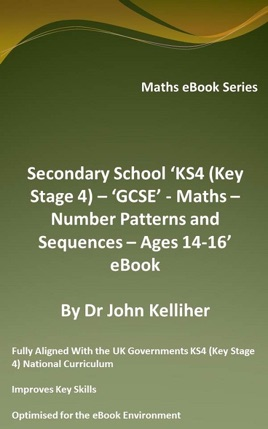• 2,99 €

## Descrizione dell’editore

This eBook again introduces the student to number patterns and sequences, including odd and even numbers, square numbers, square roots, cube numbers, cube roots, prime numbers, linear sequences, square number and cube number sequences, Fibonacci number sequences, triangular number sequences and sequences of the powers of 2, 3, 4, 5 and 10. This eBook is part of our range of Key Stage 4 (KS4) GCSE maths eBooks that are fully aligned with the UK Governments national curriculum.